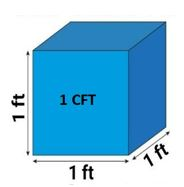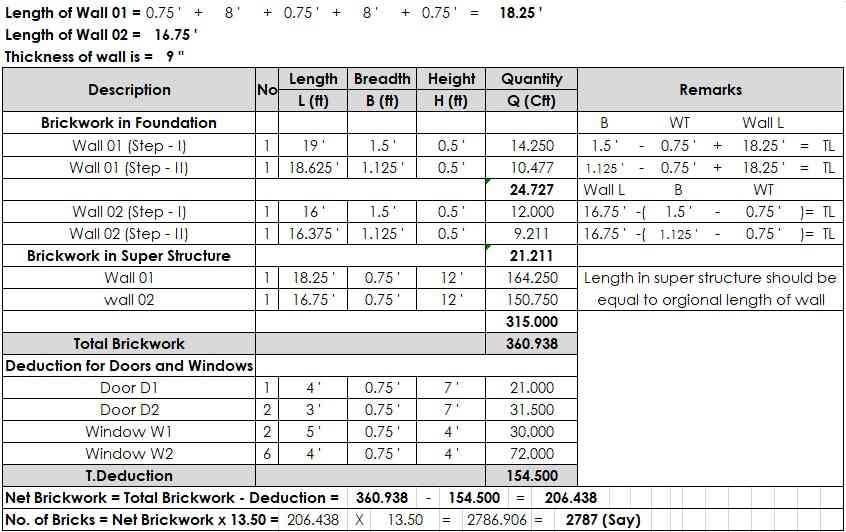# How to Calculate No of Bricks in One Cft, Wall, Room and Building

In this Article today we will talk about the How to Calculate Bricks in a Wall | Brickwork Calculation Formula | How to Calculate Bricks in a Room | Bricks per square feet | How Many Bricks in 1 Cft | Brick Calculator in feet | How to find brick quantity in wall | How to Calculate No of Bricks in feet | Calculate bricks in 9 inch wall

## How to Calculate Bricks in a Wall:

The Quantity of bricks calculation is very important because most of the buildings are to be constructed with 75% of brickwork especially (In the Asian countries). So it is very important for us to know how many bricks are required in one cubic feet volume.

After knowing the 1 Cft volume we can calculate the remaining quantity very easily my just multiplying 1 Cft result to the desired volume. Brickwork Calculation Formula

The calculation is quite simple means how many numbers of the bricks you will require to build your wall or room or building, Let us find how to calculate the number of bricks:

## How to Find Bricks in One Cft?

Length of Brick = L   = 9”  = 0.75 ft

Width of Brick = W   = 4.5”  = 0.375 ft Brickwork Calculation Formula

Thickness of Brick = T   = 3”  = 0.25 ft Volume of Brick = V  = L X W X T = 0.75 x 0.375 x 0.25                                                                        = 0.070 Cft

No. of Dry Bricks in One CFT   =  Volume of One Cft / Volume of Brick

No. of Dry Bricks in One CFT   = 1/0.070 = 14.286 Bricks (Without Mortar Space)I want to clear you guys, 14.286 Bricks without mortar in one cft, now we should deduct the space of mortar from this quantity because we are applying bricks with mortar so we deduct 10% mortar space from 14.286 Bricks.

Mortar Covered 10% Space  = 14.286 x  10/100

Mortar Covered 10% Space = 1.4286 Bricks (Mortar Space)

Quantity of Bricks with Mortar  = 14.2861.4286  = 12.857 Bricks

Adding 5% Wastage of Bricks  = 12.857 x  5/100 = 0.6428 Bricks

Hence, Brickwork Calculation Formula

Total No. of Bricks in 1 Cft  = Quantity of Bricks with Mortar + 5% Wastage of Bricks

Total No. of Bricks in 1 Cft   = 12.857 + 0.6428 = 13.4998

Total No. of Bricks in 1 Cft   = 13.50 (Say)

Hence Proved that 1 Cft = 13.50 Bricks or 100 Cft = 1350 Bricks

## How to Find Bricks in a Wall?

Let Take an example, The length of the wall = 10 ft, height = 6 ft, and thickness of the wall = 4.5 in. Calculate bricks in 9 inch wall

Length of Wall = L = 10 ft

Height of Wall = H = 6 ft

Thickness of Wall = T = 4.5 in = 4.5/12 = 0.375 ft (All units should be same in feets)

Volume of Brickwork = L x H x T = 10 x 6 x 0.375 = 22.50 Cft

We know that, for 1 Cft brickwork = 13.50 Nos required (As we calculated in above)

So, for 22.50 Cft of brick work = 22.50 x 13.50 = 303.75 = 304 Nos. (Say)

So, 10′ x 6′ x 4.5″ wall required 304 No. of Bricks Brickwork Calculation Formula

## How to Find Bricks in a Room?

### Wall 01:

Length of Wall 01 = L = 10 ft

Height of Wall 01 = H = 12 ft

Thickness of Wall 01 = T = 9″ = 9/12 = 0.75 ft How to Calculate Bricks in a Room

### Wall 02:

Length of Wall 02 = L = 11.50 ft

Height of Wall 02 = H = 12 ft

Thickness of Wall 02 = T = 9″ = 9/12 = 0.75 ft Calculate bricks in 9 inch wall

### Door:

Length of Door = L = 4 ft

Height of Door = H = 7 ft

Thickness of Door = T = 9″ = 9/12 = 0.75 ft Calculate bricks in 9 inch wall

### Window:

Length of Window = L = 5 ft

Height of Window = H = 4 ft

Thickness of Window = T = 9″ = 9/12 = 0.75 ft

### Volume of Walls:

Volume of Wall 01 = L x H x T = 10 x 12 x 0.75 = 90.00 Cft

Volume of Wall 02 = L x H x T = 11.50 x 12 x 0.75 = 103.50 Cft

Total Volume of Walls = Volume of Wall 01 + Volume of Wall 02

Total Volume of Walls = 90 + 103.50 = 193.50 Cft

### Volume for Deduction (Door + Window):

Volume of Door = L x H x T = 4 x 7 x 0.75 = 21.00 Cft

Volume of Window = L x H x T = 5 x 4 x 0.75 = 15.00 Cft

Total Volume for Deduction = Volume of Door + Volume of Window

Total Volume for Deduction = 21.00 + 15.00 = 36.00 Cft

### Net Volume:

Net Volume = Total Volume of Walls – Total Volume for Deduction

Net Volume = 193.50 – 36 = 157.50 Cft

### No. of Bricks Calculation:

No. of Bricks in one Cft = 13.50 Bricks

Total No. of Bricks = Net Volume x 13.50

Total No. of Bricks = 157.50 x 13.50 = 2126.25 Bricks = 2127 Bricks (Say)

Hence, the total No. of Bricks used in Construction of Room are 2127 Bricks

## How to Find Bricks in a Building?

Length of Wall 01 = 0.75′ + 8′ + 0.75′ + 8′ + 0.75′ = 18.25′

Length of Wall 02 = 16.75′ (As given)

Thickness of Wall = 9″ = 0.75′ (As given) Bricks per square feet

### Brickwork in Foundation:

Volume of B/W in Wall 01 (Step-I) = L x B x H = 19′ x 1.50′ x 0.50′ = 14.25 Cft (Where, Length of Wall = 1.5′ – 0.75′ + 18.75′ = 19′ for adding offset in foundation)

Volume of B/W in Wall 01 (Step-II) = L x B x H = 18.625′ x 1.125′ x 0.50′ = 10.477 Cft (Where, Length of Wall = 1.125′ – 0.75′ + 18.75′ = 18.625′ for adding offset in foundation) How to find brick quantity in wall

Total Volume of B/W in Wall 01 = 14.25 + 10.477 = 24.727 Cft

Volume of B/W in Wall 02 (Step-I) = L x B x H = 16′ x 1.50′ x 0.50′ = 12.00 Cft {Where, Length of Wall = 16.75′ – (1.50′ – 0.75′) = 16′ for subtracting offset in foundation because this offset is already included in wall 01 Step-I B/W)

Volume of B/W in Wall 02 (Step-II) = L x B x H = 16.375′ x 1.125′ x 0.50′ = 9.211 Cft (Where, Length of Wall = 16.75′ – (1.125′ – 0.75′) = 16.375′ for subtracting offset in foundation because this offset is already included in wall 01 Step-II B/W)

Total Volume of B/W in Wall 02 = 12.00 + 9.211 = 21.211 Cft Bricks per square feet

### Brickwork in Super Structure:

Volume of B/W in Wall 01 = L x B x H = 18.25′ x 0.75′ x 12′ = 164.250 Cft

Volume of B/W in Wall 02 = L x B x H = 16.75′ x 0.75′ x 12′ = 150.750 Cft

Total Volume of B/W in Walls = 164.250 + 150.750 = 315.00 Cft Bricks per square feet

### Total Brickwork in Building:

Total B/W in Building = 24.727 + 21.211 + 315.00 = 360.938 Cft Bricks per square feet

### Brickwork Deduction for Doors and Windows:

Volume of B/W in Door D1 = No x L x B x H = 1 x 4′ x 0.75′ x 7′ = 21.00 Cft

Volume of B/W in Door D2 = No x L x B x H = 2 x 3′ x 0.75′ x 7′ = 31.500 Cft

Volume of B/W in Window W1 = No x L x B x H = 2 x 5′ x 0.75′ x 4′ = 30.00 Cft

Volume of B/W in Window W2 = No x L x B x H = 6 x 4′ x 0.75′ x 4′ = 72.00 Cft

Total Deduction for Doors and Windows = 21.00 + 31.50 + 30.00 + 72.00 = 154.50 Cft Brick Calculator in feet

### Net Brickwork:

Net Brickwork in Building = Total Brickwork in Building – Deduction for Doors and Windows

Net Brickwork in Building = 360.938 – 154.50 = 206.438 Cft Brick Calculator in feet

### No. of Bricks Calculation:

No. of Bricks = Net Brickwork x 13.50 = 206.438 x 13.50 = 2786.906 = 2787 Bricks (Say) Brick Calculator in feet

Hence, Total No. of Bricks used in Building Construction are 2787

### Same Brickwork Calculation in Table:## General Formulas for Bricks and Blocks Calculation | Thumb Rules:

1. Single Brick Pier = 14 bricks per vertical metre
2. One and a Half Brick Pier = 34 bricks per vertical metre
3. Surface Area for Brickwork = Length of the Wall x Height of the Wall
4. Half-Brick thick Wall = Surface Area x 60
5. One-Brick thick Wall = Surface Area x 120
6. Single Brick Piers = Vertical Metre x 14
7. One and a Half Brick Piers = Vertical Metre x 34
8. Brick Wastage = 10% of total quantity required
9. 10% of Brickwork will be coverd by Mortar
10. 13.50 No. of Bricks in one Cft
11. 500 No. of Bricks in 1 cum
12. Standard, UK, metric blocks are roughly 450 x 215 x 100mm (some are thicker but this will not effect the number of blocks required per square metre of wall), the mortar joints used are normally about 10mm both horizontally and vertically.
13.   A single block wall requires 10 blocks per square metre.
14. So the total number of blocks for the wall is: wall height (metres) x wall length (metres) x 10 = number of blocks Brick Calculator in feet

### Example for Brickwork:

Quantity for Brick Wall: 9m2 (surface area) x 60 (half-brick thick wall) = 540 bricks

Two single Brick Piers: 1.5m (vertical height) x 14 = 42 bricks

Wastage of 10%: 58.2 bricks

Total Bricks Needed: 640.2 bricks needed How to find brick quantity in wall

### Example for Blockwork:

Wall Size = 3000 mm x 440 mm x 1500 mm

Quantity of Blocks = L x H x 10 = 3.0 x 1.50 x 10 = 45 Blocks

No of Blocks =  45 Blocks How to find brick quantity in wall

[wpdm_package id=’4868′]

## Conclusion:

Full article on How to Calculate Bricks in a Wall | Brickwork Calculation Formula | How to Calculate Bricks in a Room | Bricks per square feet | How Many Bricks in 1 Cft | Brick Calculator in feet | How to find brick quantity in wall | How to Calculate No of Bricks in feet | Calculate bricks in 9 inch wall. Thank you for the full reading of this article in “The Civil Engineering” platform in English. If you find this post helpful, then help others by sharing it on social media. If any formula of BBS is missing from this article please tell me in comments.

1.NEEL GAGAN DAS
2.Stephen Beyan Gayflorzee